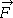• JessicaHelena

## Homework Statement

In the figure below, a chain consisting of five links, each of mass 0.103 kg, is lifted vertically with a constant acceleration of magnitude a = 2.49 m/s2.
(a) Find the magnitude of the force acting between link 1 and link 2.
(b) Find the magnitude of the force acting between link 2 and link 3.
(c) Find the magnitude of the force acting between link 3 and link 4.
(d) Find the magnitude of the force acting between link 4 and link 5.
(e) Find the magnitude of the forceexerted on the top link by the person lifting the chain.
(f) Find the magnitude of the net force accelerating each link.

## The Attempt at a Solution

I didn't quite know what I was supposed to do, so googled the problem (I'm not really trying to cheat though). I found this https://answers.yahoo.com/question/index?qid=20110322030804AAe7RWU. While I can see how to get each of those answers, I don't understand why you just keep on adding the values. Also, there are many forces acting on each link, so what does the question mean by the force "acting between" certain links? Understanding how to solve probs is more important than just solving them for me, so please help me understand why.

#### Attachments

Start by assuming your chain consists only of one link (link #1). Could you explain how to calculate the necessary force to lift it upwards with a constant acceleration of 2.49m/s^2?

... While I can see how to get each of those answers, I don't understand why you just keep on adding the values.
Think of this: you are hanging on a rope, hands don't hurt much. Now someone grabs your ankles and they are being supported by you. Do you hands feel the same?

•stockzahn
@stockzahn — That would be 2.49 * 0.103 + 0.103 * 9.8 (part a)...

@phinds — oh so each time you have one more link, you need the force needed to lift it up at the same acceleration (what stockzahn wanted me to find), and so add it...?

oh so each time you have one more link, you need the force needed to lift it up at the same acceleration (what stockzahn wanted me to find), and so add it...?
Right. Each link has to support ALL of the links below it